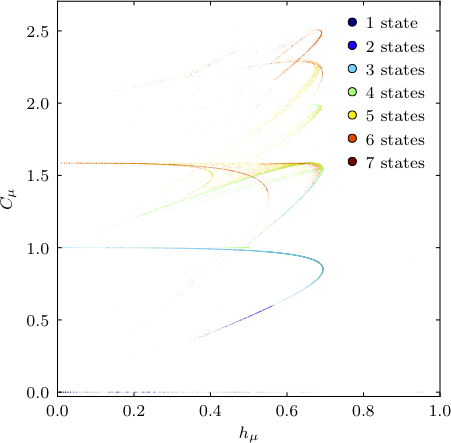# structural drift.

The structural drift project looks at sequential inference, where chains of $$\epsilon$$-machines are inferred and re-transmit data from generation to generation. A simple analogy is the game of telephone, where a person thinks of a sentence and whispers it to their neighbor, who in turn whispers it to a different neighbor, and so on. The last recipient announces the sentence they were told, which is often amusingly different from the initial sentence due to errors accumulated in the chain.It turns out that, when used in a similar way, sequential inference with $$\epsilon$$-machines is a generalization of genetic drift. For details on this connection to population genetics, see the paper. This page focuses on a series of programming examples, using the Computational Mechanics in Python library, to explore the higher level ideas presented in the paper.

# finite data inference.

To reconstruct an $$\epsilon$$-machine from data, we first generate data from a stochastic process:

data = Even().symbols(2000)
s = Splitter(data, history_length=3)
s.write('even.dot')


Here we create an even process iframe and use it to generate a binary string of length 2000 where blocks of 1s are even in length. This data is passed to the state splitting reconstruction algorithm along with the history length parameter. This is the default value of the parameter and is omitted from the remaining examples. Finally, we write the machine to a Graphviz file for viewing.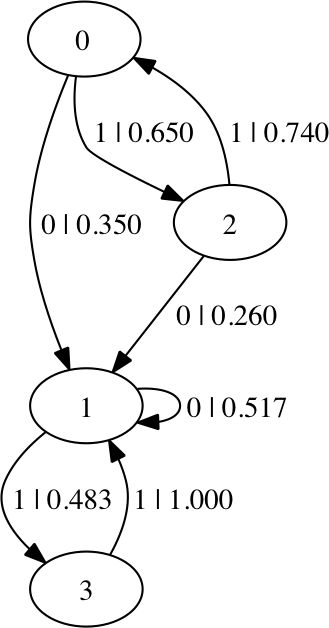# sequential inference.

Building on this example, we use the reconstructed machine to generate a new string of data having the same distribution of symbols as the original string, plus some variance due to finite sampling.

data = Even().symbols(2000)
s = Splitter(data)
s.write('even.dot')

data_new = s.symbols(2000)
s = Splitter(data_new)
s.write('even_new.dot')


The new string generated by the first machine is then fed back to the reconstruction algorithm to produce a second machine. Each machine is written to a separate Graphviz file for comparison, and should have identical topologies with slightly different transition probabilities. The machines are omitted here for space.

The next step is to iterate this chain of inference, generation, and re-inference until some condition occurs. For now we’ll say this terminating condition is when there are no more 0s generated by the machine, which can occur by chance.

zero = tuple(['0'])

data_length = 2000
data = Even().symbols(data_length)
s = Splitter(data)
p_zero = count_blocks(data, probabilities=True)[zero]

while p_zero > 0:
data = s.symbols(data_length)
s = Splitter(data)
p_zero = count_blocks(data, probabilities=True)[zero]

s.write('even.dot')


We introduce the count_blocks function which returns a dictionary mapping blocks of symbols to probabilities, normalized for each block length. Symbols are stored as tuples since lists are not hashable in Python, and so we create the variable zero for convenience.

The code will loop until there are no more 0s in the data due to the cumulative effects of finite sampling variance. The machine could also drift the other way by chance and produce only 0s, but this is less likely for the even process. Therefore, the final inferred machine is typically a fixed coin that only generates 1s.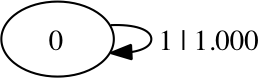# structural stasis.

It’s easy to decide when the inference loop should terminate for the simple even process. For more complex processes, however, a more general condition is needed to detect when the structure of the machine can no longer drift.

We call this condition structural stasis, and it occurs when the entropy rate of the machine ($$h_\mu$$) becomes zero. We modify the above example to reflect our definition of structural stasis, and plot the drift of entropy rate as a function of generation.

data_length = 1000
data = Even().symbols(data_length)
s = Splitter(data)
entropy_rates = []

while s.entropy_rate() > 0:
data = s.symbols(data_length)
s = Splitter(data)
entropy_rates.append(s.entropy_rate())

print 'stasis at generation', len(entropy_rates)

pylab.plot(entropy_rates)
pylab.xlabel('Generation')
pylab.ylabel('$h_u$')
pylab.savefig('even.pdf')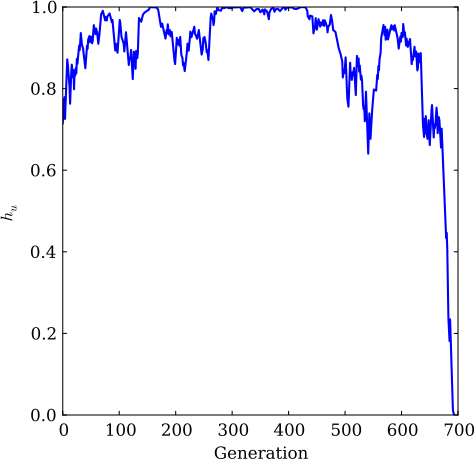By setting a low initial bias for the 0-transition of the even process, we can see how the number of generations until stasis is affected. This is achieved by passing the bias=0.05 parameter to the even process constructor.

data_length = 1000
data = Even(bias=0.05).symbols(data_length)
s = Splitter(data)
entropy_rates = []

while s.entropy_rate() > 0:
data = s.symbols(data_length)
s = Splitter(data)
entropy_rates.append(s.entropy_rate())

pylab.plot(entropy_rates)
pylab.xlabel('Generation')
pylab.ylabel('$h_u$')
pylab.savefig('drift_even_bias05.pdf')


While this “time to stasis” is for a single realization, the result below holds true when averaged over many different experiments: the closer a process starts to a fixed point, the shorter the average time to stasis.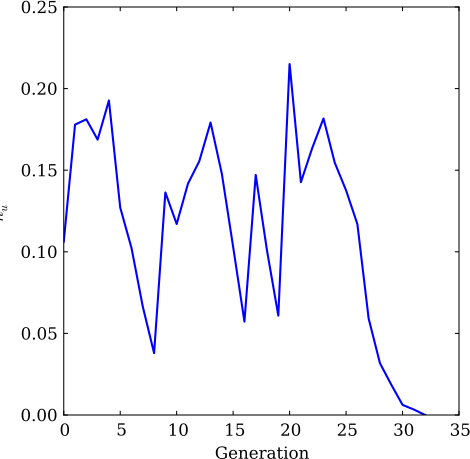# complexity-entropy diagrams.

Another observation from the paper is that machines tend to diffuse locally in a stable subspace until enough drift variance has accumulated to create or merge states. As a result, the process changes topology and jumps to a new subspace. These subspaces are independent, and so the time to stasis for a process is a weighted sum over the stasis times for the independent subspaces the drifting machines visit on their way to reaching stasis.

data_length = 1000
data = GoldenMean().symbols(data_length)
s = Splitter(data)
entropy_rates = []
statistical_complexities = []

while s.entropy_rate() > 0:
data = s.symbols(data_length)
s = Splitter(data)
entropy_rates.append(s.entropy_rate())
statistical_complexities.append(s.statistical_complexity())

pylab.plot(entropy_rates, statistical_complexities, '.', markersize=1.0)
pylab.ylim([-0.1, 1.1])
pylab.xlabel('$h_u$')
pylab.ylabel('$C_u$')
pylab.savefig('ce_gm.pdf')


One way to examine these subspaces is a complexity-entropy (CE) diagram, which plots the entropy rate of a machine ($$h_u$$) versus its statistical complexity ($$C_u$$). The CE diagram of a single realization of the golden mean process, producing binary strings with no consecutive 0s, is shown below. The upper curve shows diffusion in the golden mean subspace, and the line $$C_u=0$$ shows diffusion in the biased coin subspace after the two recurrent states of the golden mean are merged due to drift.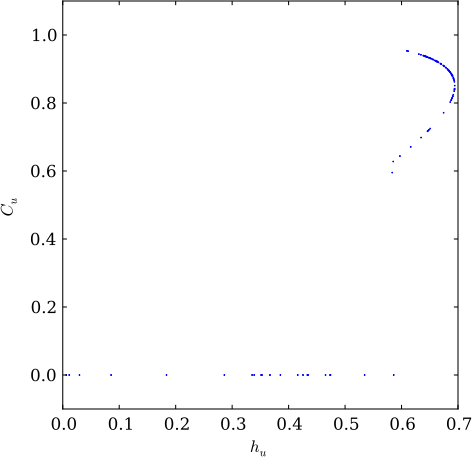The butterfly process has a more complex CE diagram, and is obtainable by using a Butterfly object in the above code and setting data_length=15000.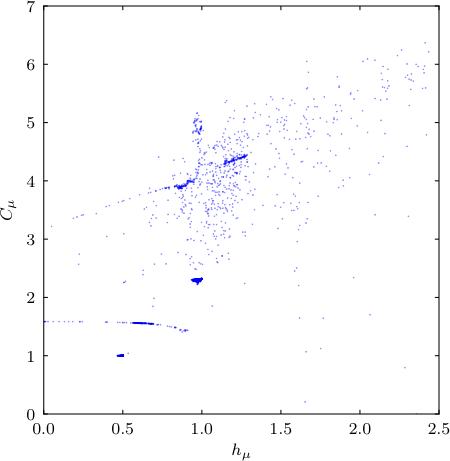# isostructural subspaces.

We see a different view of the golden mean drift process by reducing the evidence needed to infer new states and showing the total number of states as colored points in CE space. This shows that machines locally diffuse within each subspace leading to meta-stability of the drift process, with occasional jumps to new subspaces as the model’s memory dynamically adjusts to the statistics of the new iteration: